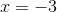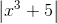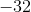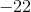## Example Questions

### Example Question #1 : How To Find Absolute Value

Quantitative Comparison:

Column A

|–3 + 4|

Column B

|–3| + |4|

Cannot be determined

Column A and B are equal

Column A is greater

Column B is greater

Column B is greater

Explanation:

The operations in the absolute value are always done first. So in Column A, |–3 + 4| = |1| = 1.  In Column B, |–3| + |4| = 3 + 4 = 7.

### Example Question #1 : How To Find Absolute Value

Quantitative Comparison

|x – 3| = 3

Quantity A: x

Quantity B: 2

The two quantities are equal.

The relationship cannot be determined from the information given.

Quantity B is greater.

Quantity A is greater.

The relationship cannot be determined from the information given.

Explanation:

It's important to remember that absolute value functions yield two equations, not just one. Here we have x – 3 = 3 AND x – 3 = –3.

Therefore x = 6 or x = 0, so the answer cannot be determined.

If we had just used the quation x – 3 = 3 and forgotten about the second equation, we would have had x = 6 as the only solution, giving us the wrong answer.

### Example Question #1 : Absolute Value

Quantitative Comparison

Quantity A: |10| – |16|

Quantity B: |1 – 5| – |3 – 6|

The relationship cannot be determined from the information given.

The two quantities are equal.

Quantity A is greater.

Quantity B is greater.

Quantity B is greater.

Explanation:

Quantity A: |10| = 10, |16| = 16, so |10| – |16| = 10 – 16 = –6.

Quantity B: |1 – 5| = 4, |3 – 6| = 3, so |1 – 5| - |3 – 6| = 4 – 3 = 1.

1 is bigger than –6, so Quantity B is greater.

### Example Question #2 : Absolute Value

Quantitative Comparison

Quantity A: (|–4 + 1| + |–10|)2

Quantity B: |(–4 + 1 – 10)2|

Quantity B is greater.

The relationship cannot be determined from the information given.

Quantity A is greater.

The two quantities are equal.

The two quantities are equal.

Explanation:

Quantity A: |–4 + 1| = |–3| = 3 and |–10| = 10, so (|–4 + 1| + |–10|)2 = (3 + 10)2 = 13= 169

Quantity B: |(–4 + 1 – 10)2| = |(–13)2| = 169

The two quantities are equal.

### Example Question #1 : Absolute Value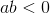Quantity A: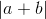Quantity B: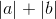Quantity B is greater

Quantity A is greater

The relationship cannot be determined from the information given

The two quantities are equal

Quantity B is greater

Explanation:

If, then eitherormust be negative, but not both. Making them both positive, as in quantity B, and then adding them, would produce a larger number than adding them first and making the result positive.

### Example Question #5 : How To Find Absolute Value

What is the absolute value of the following equation when